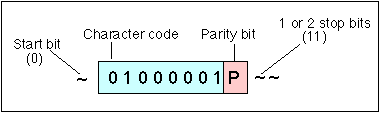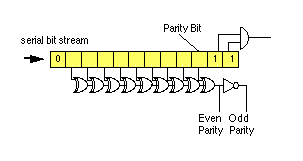# Character Parity

Parity is probably the simplest form of Integrity Check.

Character or byte parity is an error detection technique that is typically used on asynchronous links to provide a per-byte integrity check.

It is used to verify the integrity of individual received bytes. When used, each character is protected at the sender by adding a single parity baud (or bit) to each character (byte) that is transmitted. This is sent after the data bits/bauds.

The value of the parity bit is calculated by performing a logical exclusive OR (XOR) that combines all of the bits in the byte. This calculation is often peformed in hardware by the UART, as a part of the transmission function. Two types of parity are used:

• Even parity where a 1-parity-bit is sent if there are an even number of 1-bits
• Odd parity which is the opposite (i.e. a 1-parity-bit is sent when there are an odd number of 1-bits).

A receiver needs to be configured to use the type of parity that is used (if any) by the sender. When each byte (character) has been received, a parity value is accumulated (XOR-ing all data bits) using the same algorithm as the sender. The receiver then compares the receiver-calculated parity value with the receieved value in the parity baud. There are two outcomes possible:

• If the two values match, the byte is accepted as valid.
• If the two values do not match, then there must have been a transmission error. This error may for instance set an error flag in the status word of a UART, causing the receiver to abort reception.Parity baud added to a transmitted character at the transmitter and checked at the receiver

The parity value may be calculated using either an exclusive-or adder or a Finite State Machine (FSM). An implementation using XOR gates is shown below:Hardware to Implement a Parity Checker

As an integrity check, a simple byte parity is relatively weak. It is sufficient to be used as a simple transmission check, but is unable to detect any even number of bit errors (which would result in the same parity value). To overcome these limitations, many systems combine this with a per-frame checksum (such as longitudinal parity) or replace the checksum with a more powerful Cyclic Redundancy Check (CRC).

## Example Using Parity

Parity may also be calculated in software using a shift register to count the number of '1' bits in each byte. When the calculated parity from the received character does not match the value of the received parity bit, then a parity error is said to have occurred, and the character is normally discarded. This parity check detects any number of odd errors but passes any number of even errors without detecting an error.

Here are some examples to check your understanding. A message "Hello" was sent to a serial command using the C programming statement "printf("Hello\n").

The original sequence of characters are shown in column 1.

The binary bit patterns which are sent are shown in the second column (use an ASCII table to see if this is represented in computer bit order or transmission bit order!)

The third column shows the sequence of received bits, including some transmission errors (can you identify which bits were corrupted?).

The received ASCII characters and the parity condition are shown in the final columns. (Can you validate that the parity logic detects these errors?)

```H S010010011SS S010010011SS H Valid 'H'
e S011001010SS S011011010SS ¿ Parity Error
l S011011000SS S0110110000S ¿ Invalid
l S011011000SS S011111001SS v Valid 'v'
o S011011110SS S011011110SS o Valid 'o'
cr S000011011SS S111011011SS ¿ Parity Error```

Parity, is good at detecting single errors, and will always detect any odd number of errors in a received character. If, however, there are an even number of errors, the parity checker will be unable to find the error. Consider the transmission of the same message and the received sequence below. Can you spot a line which contains a good parity value but actually contains some errors?

##### Receive (second pattern of errors):
```S010010011SS
S011001010SS
S011011000SS
S011011000SS
S011011110SS
S000011011SS

```

## Longitudinal Parity

Parity can also be used across the length of a block of data to perform an integrity check. In this way, the check can help detect errors, especially when a parity baud is also added to bytes of data. A more sophsiticated check can be performed by calculating a Cyclical Redundancy Check, CRC.